Next: Example Syzygy Calculations Up: Lunar-Solar Syzygies and Eclipses Previous: Introduction

# Determination of Lunar-Solar Elongation

We can determine the lunar-solar elongation by combining the solar and lunar models described in the previous two chapters. Our elongation model is as follows: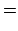(133)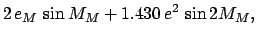(134)(135)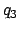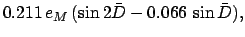(136)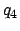(137)(138)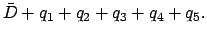(139)

Here,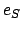,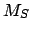, and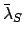are the eccentricity, mean anomaly, and mean longitude of the sun's apparent orbit about the earth, respectively. Moreover,,,, andare the eccentricity, mean anomaly, mean longitude, and mean argument of latitude of the moon's orbit, respectively.

The lunar-solar elongation can be calculated with the aid of Tables 40 and 41. Table 40 allows the mean lunar-solar elongation,, the mean lunar argument of latitude,, the mean anomaly of the sun,, and the mean anomaly of the moon,, to be determined as functions of time. Table 41 specifies the anomalies-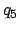as functions of their various arguments.

The procedure for using the tables is as follows:

1. Determine the fractional Julian day number,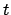, corresponding to the date and time at which the lunar-solar elongation is to be calculated with the aid of Tables 27-29. Form, where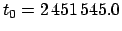is the epoch.
2. Enter Table 40 with the digit for each power of 10 in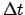and take out the corresponding values of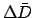,,, and. Ifis negative then the values are minus those shown in the table. The value of the mean lunar-solar elongation,, is the sum of all thevalues plus the value ofat the epoch. Likewise, the value of the mean lunar argument of latitude,, is the sum of all thevalues plus the value ofat the epoch. Moreover, the value of the solar mean anomaly,, is the sum of all thevalues plus the value ofat the epoch. Finally, the value of the lunar mean anomaly,, is the sum of all thevalues plus the value ofat the epoch. Add as many multiples ofto,,, andas is required to make them all fall in the rangeto.
3. Form the five arguments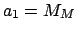,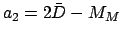,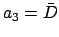,,. Add as many multiples ofto the arguments as is required to make them all fall in the rangeto. Round each argument to the nearest degree.
4. Enter Table 41 with the value of each of the five arguments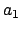-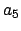and take out the value of each of the five corresponding anomalies-. It is necessary to interpolate if the arguments are odd.
5. The lunar-solar elongation is given by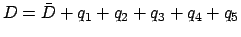. If necessary, convertinto an angle in the rangeto. The decimal fraction can be converted into arc minutes using Table 31.

In order to facilitate the calculation of syzygies, the above model has been used to contruct Table 42, which lists the dates and fractional Julian day numbers of the first new moons of the years 1900-2099 CE. Two examples of syzygy calculations are given below.Next: Example Syzygy Calculations Up: Lunar-Solar Syzygies and Eclipses Previous: Introduction
Richard Fitzpatrick 2010-07-21